# Wet Gas Correction Factors

## Condensate Density

The condensate density is required when calculating the total liquid density. The next five steps are required to calculate the condensate density. If the configuration option -

Condensate Stream Density - User Input

is selected this is skipped and the user entered value is used instead.

## Compressibility Factor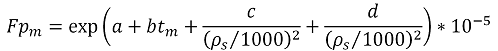Symbol Description Units
Fpm Compressibility factor -
tm Stream temperature °C
ρs Condensate standard density kg/Sm3

Constant Value
a -1.62080
b 0.00021592
c 0.87096
d 0.0042092

## Condensate Pressure Correction Factor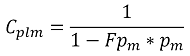Symbol Description Units
Cplm Condensate pressure correction factor -
Fpm Compressibility factor -
pm Stream pressure bar g

## Coefficient of Thermal Expansion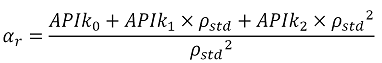tr>
Symbol Description Units
αr Coefficient of thermal expansion -
ρstd Standard condensate density kg/Sm3
APIk0 From calibration certificate -
APIk1 From calibration certificate -
APIk2 From calibration certificate -

## Temperature Correction Factor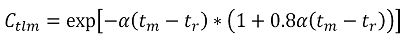Symbol Description Units
Ctlm Temperature correction factor -
α Coefficient of thermal expansion -
tm Stream temperature °C
ts Standard temperature °C

## Condensate Density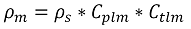Symbol Description Units
ρm Condensate density kg/m3
ρs Condensate standard density kg/Sm3
Cplm Condensate pressure correction factor -
Ctlm Condensate temperature correction factor -

## Water Density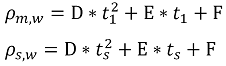Symbol Description Units
ρm,w Water stream density kg/m3
t1 Gas upstream temperature °C
ρs,w Water standard density kg/Sm3
ts Gas standard temperature °C

Constant Value
D -0.0001732
E -0.1307
F 1040

## Methanol Density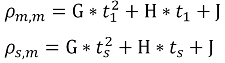Symbol Description Units
ρm,m Methanol stream density kg/m3
t1 Gas upstream temperature °C
ρs,m Methanol standard density kg/Sm3
ts Gas standard temperature °C

Constant Value
G 7.13E-05
H -0.3344
J 540

## Liquid to Gas Ratio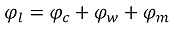Symbol Description Units
φl Liquid to gas mass ratio -
φc Operator entered condensate to gas mass ratio -
φw Operator entered water to gas mass ratio -
φm Operator entered methanol to gas mass ratio -

## Wet Gas Mass Fractions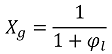Symbol Description Units
Xg Wet gas mass fraction -
φl Liquid to gas mass ratio -

## Liquid Component Mass Fractions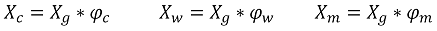Symbol Description Units
Xg Wet gas mass fraction -
Xc Condensate mass fraction -
Xw Water mass fraction -
Xm Methanol mass fraction -
φc Operator entered condensate to gas mass ratio -
φw Operator entered water to gas mass ratio -
φm Operator entered methanol to gas mass ratio -

## Liquid Density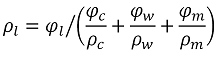Symbol Description Units
ρl Liquid density kg/m3
ρc Condensate stream density kg/m3
ρw Water stream density kg/m3
ρm Methanol stream density kg/m3
φl Liquid to gas mass ratio -
φc Operator entered condensate to gas mass ratio -
φw Operator entered water to gas mass ratio -
φm Operator entered methanol to gas mass ratio -

## Lockhart-Martinelli Factor - Orifice Meter

The following calculation is carried out if any correction factor other than the Steven correction factor is selected.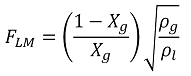Symbol Description Units
FLM Lockhart-Martinelli factor -
Xg Gas mass fraction -
ρg Gas density kg/m3
ρl Liquid density kg/m3

## Froude Number - Orifice Meter

The following calculation is carried out if either the Chisholm or De Leeuw correction factor is selected.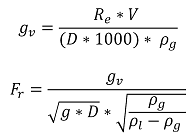Symbol Description Units
Fr Froude Number -
gv Gas velocity -
Re Reynolds Number -
V Fluid viscosity Cp
D Corrected pipe diameter m
ρg Gas density kg/m3
ρl Liquid density kg/m3

Gravitational Constant Value
g 9.80664999999999

## Murdock Correction Factor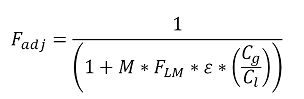Symbol Description Units
Fadj Murdock correction factor -
M Operator entered Murdock Factor(default = 1.26) -
FLM Lockhart Martinelli factor -
ε Gas expansion factor -
Cg Gas coefficient of discharge -
Cl Liquid coefficient of discharge -

## Chisholm Correction Factor

If the Froude Number is below 0.5 the Chisholm correction factor = 1.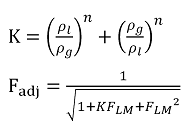Symbol Description Units
Fadj Chisholm correction factor -
FLM Lockhart Martinelli factor -
ρl Liquid density kg/m3
ρg Gas density kg/m3
n Operator entered Chisholm Factor(default = 0.25) -

## De Leeuw Correction Factor

For Froude Numbers above 1.5: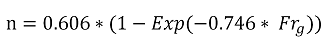For Froude Numbers below 1.5: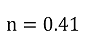De Leeuw CorrectionSymbol Description Units
Fadj De Leeuw correction factor -
Frg Froude Number -
FLM Lockhart Martinelli factor -
ρl Liquid density kg/m3
ρg Gas density kg/m3
n De Leeuw Factor -

## Steven Correction Factor (The following steps are only carried out when the 'Steven Factor' is selected.)

Because the corrected mass flow rate is used to calculate the Steven correction factor an iterative method is required. The following calculations iterate up till the previous mass flowrate less than 0.00001 out from the current mass flowrate or a maximum of 100 iterations is reached.

## Inlet Area of the V-Cone Meter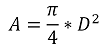Symbol Description Units
A Inlet area of the v-cone meter at operating conditions m2
D Internal pipe diameter at working conditions m

## Liquid Mass Flowrate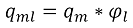Symbol Description Units
qml Liquid mass flowrate kg/s
qm Gas corrected mass flowrate kg/s
φl Liquid to gas mass ratio -

## Lockhart-Martinelli Factor

For configuration option: Include Clamping - Yes
If the density ratio is less than 0.027 the ratio is clamped to 0.027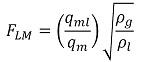Symbol Description Units
FLM Lockhart-Martinelli factor -
qml Liquid mass flowrate kg/s
qm Gas corrected mass flowrate kg/s
ρg Gas density kg/m3
ρl Liquid density kg/m3

## Superficial Gas Velocity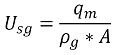Symbol Description Units
Usg Superficial gas velocity m/s
qm Uncorrected mass flowrate kg/s
ρg Gas density kg/m3
A Inlet area of the v-cone meter at operating conditions m2

## Froude Number - Cone Meter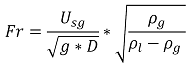Symbol Description Units
Fr Froude Number -
D Corrected pipe diameter m
ρg Gas density kg/m3
ρl Liquid density kg/m3
Usg Superficial gas velocity -

Gravitational Constant Value
g 9.80664999999999

## Wet Gas Correlation Factor A

For configuration option: Include Clamping - Yes
If the density ratio is less than 0.027 the ratio is clamped to 0.027

If the entered beta ratio is less than 0.65 the calculation for A0.55 is used

If the entered beta ratio is more than 0.65 the calculation for A0.75 is used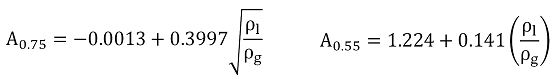Symbol Description Units
A0.55 Correlation factor for a 0.55 Beta V-Cone meter -
A0.75 Correlation factor for a 0.75 Beta V-Cone meter -
ρl Liquid density kg/m3
ρg Gas density kg/m3

## Wet Gas Correlation Factor B

For configuration option: Include Clamping - Yes
If the density ratio is less than 0.027 the ratio is clamped to 0.027

If the entered beta ratio is less than 0.65 the calculation for A0.55 is used

If the entered beta ratio is more than 0.65 the calculation for A0.75 is used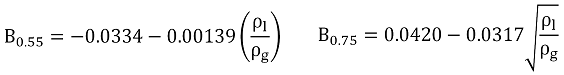Symbol Description Units
A0.55 Correlation factor for a 0.55 Beta V-Cone meter -
A0.75 Correlation factor for a 0.75 Beta V-Cone meter -
ρl Liquid density kg/m3
ρg Gas density kg/m3

## Wet Gas Correlation Factor C

For configuration option: Include Clamping - Yes
If the density ratio is less than 0.027 the ratio is clamped to 0.027

If the entered beta ratio is less than 0.65 the calculation for A0.55 is used

If the entered beta ratio is more than 0.65 the calculation for A0.75 is used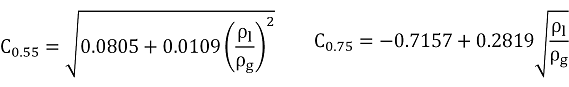Symbol Description Units
A0.55 Correlation factor for a 0.55 Beta V-Cone meter -
A0.75 Correlation factor for a 0.75 Beta V-Cone meter -
ρl Liquid density kg/m3
ρg Gas density kg/m3

## Steven Correction Factor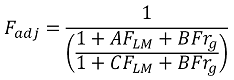Symbol Description Units
Fadj Steven correction factor -
FLM Lockhart-Martinelli factor -
Frg Froude number -
A Wet gas correlation factor -
B Wet gas correlation factor -
C Wet gas correlation factor -

## Corrected Mass Flowrate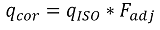Dependant on users selection the wet gas correction factor could be the Steven Correction Factor, the Murdock Correction Factor, the Chisholm Correction Factor or the De Leeuw Correction Factor.

Symbol Description Units
qcorr Corrected mass flowrate kg/s
qISO Uncorrected mass flowrate -
Fadj Wet gas correction factor -

# References

• Chisholm, D., Two phase flow through sharp-edged orifices, research note, J.Mech. Eng. Sci., 1977
• Murdock, J. W., Two phase flow measurement with orifices, J. Basic Eng., December 1962
• Steven, R., A Discussion on Horizontal Wet Gas Differential Pressure Flow Meters., 2007
• De Leeuw, H., Liquid Correction of Venturi Meter Readings in Wet Gas Flow, Paper 21., 1997
• Report on the Development, Construction, Calculation and Preperation of the ASTM-IP Petroleum Measurment Tables (ASTM D1250; IP 200), First Edition 1960 (Reprinted 1962 and 1968)
• API Manual of Petroleum Measurement Standards Chapter 11.2.2M - Compressibility Factors for Hydrocarbons: 350-637 Kilograms per Cubic Meter Density (15°C) and -46°C to 60°C Metering Temperature, 1986
• ASTM-IP-API, Petroleum Measurement Tables for Light Hydrocarbon Liquids, Density Range 0.500 to 0.653 kg/litre at 15°C, 1986